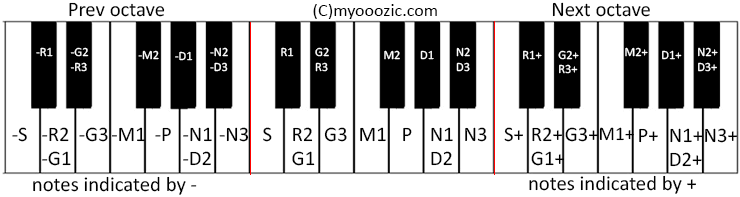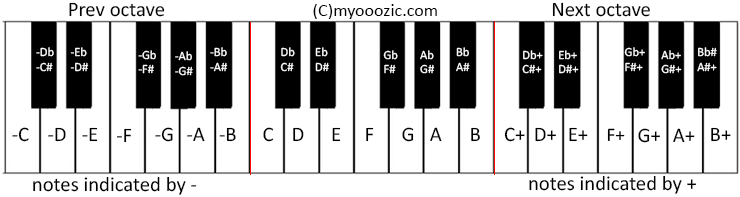# Music note for song dil mein tujhe bithake

#### Jimsweb

##### Super Moderator
Carnatic Music note for song dil mein tujhe bithake

Movie : Fakira (1976)
Singer : Lata Mangeshkar
Music : Ravindra Jain
Lyricists : Ravindra Jain
Cast : Sashi Kapoor & Shabana Azmi
Director : C P Dixit

Carnatic notation legends used for this song:Code:
``````Dil Mein Tujhe Bithaake,
d2s+r2+ s+r2+ s+pd2 d2p

Kar Lungi Main Band Aankhen
d2s+r2+r2+ s+r2+ s+d2 pd2 d2p

Pooja Karungi Teri, Ho Ke Rahungi Teri
r2p ppm1 m1m1g3r2, r2ps+d2pm1m1

Dil Mein Tujhe Bithaake,
d2s+r2+ s+r2+ s+pd2 d2p

Kar Lungi Main Band Aankhen
d2s+r2+r2+ s+r2+ s+d2 pd2 d2p

Pooja Karungi Teri, Ho Ke Rahungi Teri
r2p ppm1 m1m1g3r2, r2ps+d2pm1m1

Main Hi Main Dekhu Tujhe Piya Aur Na Dekhe Koi
d2s+pd2  s+s+ s+s+  s+ n3 n3s+r2+ s+pd2d2

Main Hi Main Dekhu Tujhe Piya Aur Na Dekhe Koi
d2s+pd2  s+s+ s+s+  s+ n3 n3s+r2+ s+pd2d2

Ek Pal Bhi Ye Soch Rahe Na Kis Vidhi Milna Hoi
d2s+r2+m1+m1+ g3+r2+  d2s+r2+g3+g3+r2+  pn3s+r2+ g3+r2+s+n3d2

Sabse Tumhen Bachaake,
d2s+r2+ s+r2+ s+pd2 d2p

Kar Lungi Main Band Aankhen
d2s+r2+r2+ s+r2+ s+d2 pd2 d2p

Pooja Karungi Teri, Ho Ke Rahungi Teri
r2p ppm1 m1m1g3r2, r2ps+d2pm1m1

Na Koi Bandhan Jagat Ka Koi Pahara Na Deevaar
d2s+d2p s+s+ s+ s+s+ s+  s+ n3 n3n3s+r2+ s+d2pd2

Na Koi Bandhan Jagat Ka Koi Pahara Na Deevaar
d2s+d2p s+s+ s+ s+s+ s+  s+ n3 n3n3s+r2+ s+d2pd2

Koi Na Jaane Do Deewane Ji Bhar Kar Le Pyaar
d2s+r2+m1+m1+ g3+r2+  d2s+r2+g3+g3+r2+  pn3s+r2+ g3+r2+s+n3d2

d2s+r2+ s+r2+ s+pd2 d2p

Tera Hi Mukh Dekh Ke Piya Raat Ko Main So Jaaun
d2s+pd2 s+s+ n3s+s+   s+ n3 n3s+r2+ s+d2pd2d2

Tera Hi Mukh Dekh Ke Piya Raat Ko Main So Jaaun
d2s+pd2 s+s+ n3s+s+   s+ n3 n3s+r2+ s+d2pd2d2

Bhor Bhai Jab Aankh Khule To Tere Hi Darshan Paaun
d2s+r2+m1+m1+ g3+r2+  d2s+r2+g3+g3+r2+  pn3s+r2+ g3+r2+s+n3d2

Tujhako Gale Lagaake,
d2s+r2+ s+r2+ s+pd2 d2p

Kar Lungi Main Band Aankhen
d2s+r2+r2+ s+r2+ s+d2 pd2 d2p

Pooja Karungi Teri, Ho Ke Rahungi Teri
r2p ppm1 m1m1g3r2, r2ps+d2pm1m1

Dil Mein Tujhe Bithaake,
d2s+r2+ s+r2+ s+pd2 d2p

Kar Lungi Main Band Aankhen
d2s+r2+r2+ s+r2+ s+d2 pd2 d2p

Pooja Karungi Teri, Ho Ke Rahungi Teri
r2p ppm1 m1m1g3r2, r2ps+d2pm1m1

Kar Lungi Main Band Aankhen
d2s+r2+r2+ s+r2+ s+d2 pd2 d2p

Pooja Karungi Teri, Ho Ke Rahungi Teri
r2p ppm1 m1m1g3r2, r2ps+d2pm1m1``````

#### Jimsweb

##### Super Moderator
Western Music note for song dil mein tujhe bithake

Western notation legends used for this song:Code:
``````Dil Mein Tujhe Bithaake,
AC+D+ C+D+ C+GA AG

Kar Lungi Main Band Aankhen
AC+D+D+ C+D+ C+A GA AG

Pooja Karungi Teri, Ho Ke Rahungi Teri
DG GGF FFED, DGC+AGFF

Dil Mein Tujhe Bithaake,
AC+D+ C+D+ C+GA AG

Kar Lungi Main Band Aankhen
AC+D+D+ C+D+ C+A GA AG

Pooja Karungi Teri, Ho Ke Rahungi Teri
DG GGF FFED, DGC+AGFF

Main Hi Main Dekhu Tujhe Piya Aur Na Dekhe Koi
AC+GA  C+C+ C+C+  C+ B BC+D+ C+GAA

Main Hi Main Dekhu Tujhe Piya Aur Na Dekhe Koi
AC+GA  C+C+ C+C+  C+ B BC+D+ C+GAA

Ek Pal Bhi Ye Soch Rahe Na Kis Vidhi Milna Hoi
AC+D+F+F+ E+D+  AC+D+E+E+D+  GBC+D+ E+D+C+BA

Sabse Tumhen Bachaake,
AC+D+ C+D+ C+GA AG

Kar Lungi Main Band Aankhen
AC+D+D+ C+D+ C+A GA AG

Pooja Karungi Teri, Ho Ke Rahungi Teri
DG GGF FFED, DGC+AGFF

Na Koi Bandhan Jagat Ka Koi Pahara Na Deevaar
AC+AG C+C+ C+ C+C+ C+  C+ B BBC+D+ C+AGA

Na Koi Bandhan Jagat Ka Koi Pahara Na Deevaar
AC+AG C+C+ C+ C+C+ C+  C+ B BBC+D+ C+AGA

Koi Na Jaane Do Deewane Ji Bhar Kar Le Pyaar
AC+D+F+F+ E+D+  AC+D+E+E+D+  GBC+D+ E+D+C+BA

AC+D+ C+D+ C+GA AG

Tera Hi Mukh Dekh Ke Piya Raat Ko Main So Jaaun
AC+GA C+C+ BC+C+   C+ B BC+D+ C+AGAA

Tera Hi Mukh Dekh Ke Piya Raat Ko Main So Jaaun
AC+GA C+C+ BC+C+   C+ B BC+D+ C+AGAA

Bhor Bhai Jab Aankh Khule To Tere Hi Darshan Paaun
AC+D+F+F+ E+D+  AC+D+E+E+D+  GBC+D+ E+D+C+BA

Tujhako Gale Lagaake,
AC+D+ C+D+ C+GA AG

Kar Lungi Main Band Aankhen
AC+D+D+ C+D+ C+A GA AG

Pooja Karungi Teri, Ho Ke Rahungi Teri
DG GGF FFED, DGC+AGFF

Dil Mein Tujhe Bithaake,
AC+D+ C+D+ C+GA AG

Kar Lungi Main Band Aankhen
AC+D+D+ C+D+ C+A GA AG

Pooja Karungi Teri, Ho Ke Rahungi Teri
DG GGF FFED, DGC+AGFF
Kar Lungi Main Band Aankhen
AC+D+D+ C+D+ C+A GA AG

Pooja Karungi Teri, Ho Ke Rahungi Teri
DG GGF FFED, DGC+AGFF``````